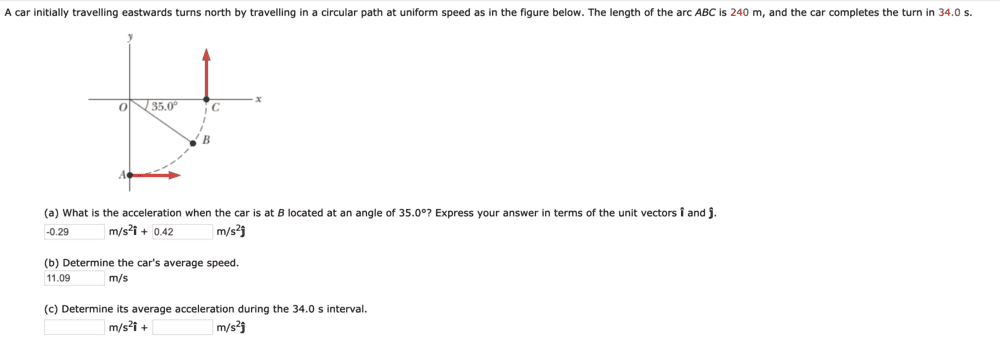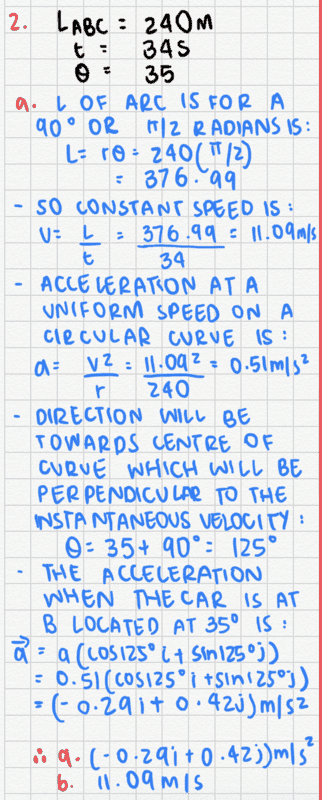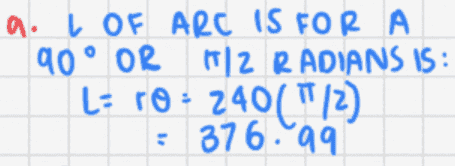# Calculating average acceleration during a time interval

Homework Statement:
A car initially travelling eastwards turns north by travelling in a circular path at uniform speed as in the figure below. The length of the arc ABC is 240 m, and the car completes the turn in 34.0 s.
(a) What is the acceleration when the car is at B located at an angle of 35.0°? Express your answer in terms of the unit vectors î and ĵ.
(b) Determine the car's average speed.
(c) Determine its average acceleration during the 34.0 s interval.

I've calculated (a) and (b) but not sure how to attempt (c)
Relevant Equations:
a=v^2/r
v=l/tHere's my calculations for (a) and (b)BvU
Homework Helper
(c) Determine its average acceleration during the 34.0 s interval.
not sure how to attempt (c)

Relevant Equations:: a=v^2/r, v=l/t
Hi,
Actually, your relevant equations give a hint: you provide one equation for the instantaneous acceleration (during a circular trajectory at constant speed) and one for the average speed (over a straight section).

If you can work around the expression $$v_{average} = {\Delta {\sf position}\over \Delta \sf time}$$(bearing in mind that position is a vector !) to something similar for average acceleration, you are in business

BvU
Homework Helper
A question:If the problem statement says
The length of the arc ABC is 240 m
why do you not believe that and calculate something else ?

A question:
View attachment 258441
If the problem statement sayswhy do you not believe that and calculate something else ?
thank you for your response! I don't understand what you mean by this.

jbriggs444
Homework Helper
It is much more convenient to respond when your work is typed in. It is then possible to quote it. The same applies for the problem statement.

We are told that "The length of arc ABC is 240 meters". You have calculated:

re-typed by hand said:
a. L of arc is for A 90 degrees or pi/2 radians is: ##L=r_0 = 240\frac{\pi}{2}=376.99##

So constant speed is ##v = \frac{L}{t} = \frac{376.99}{34} = 11.09 \text{m}/\text{s}##
That would be correct if what we were given were a radius. But we were given an arc length.

Merlin3189
Homework Helper
Gold Member
In case it helps, I decoded this for my self,
a. L of arc is for A 90 degrees or pi/2 radians is: ##L=r_0 \frac {\pi}{2} = 376.99 \, \,\, \, \text { So constant speed is }v = \frac{L}{t} = \frac{376.99}{34} = 11.09 m/s ##
That would be correct if what we were given were a radius. But we are given an arc length.

What seems to have happened: you were told the arc length but not the radius.
You thought it was the radius and calculated the corresponding arc length.
You needed to do the opposite - take their arc length and find the radius.

•jbriggs444